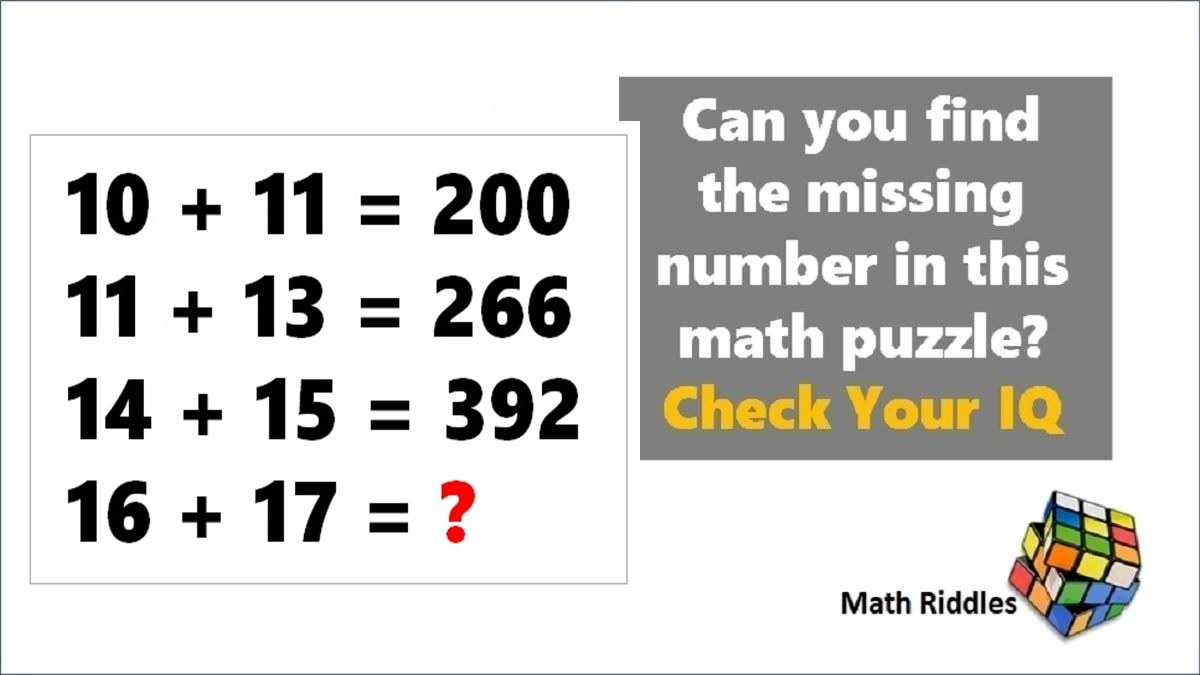# Math Riddles: 5-Minute Maths Puzzles, Find the Answers in Less than 1 Minute

Math Riddles Challenge: You are 100% genius if you can find the logic and solve this mathematics puzzle in less than 1 minute each?Math Riddles: 5-Minute Maths Puzzles, Find the Answers in Less than 1 Minute

Math Riddles: Check out these 5-minute mathematics puzzles that only 1% genius people can find the solution. Can you solve them correctly in less than 1 minute each? You are a 100% genius if you can solve these math problems. Let us begin some brain exercises.

## Solution

Explanation:

### Maths Logic Puzzle #2

Explanation:

The logic in this mathematics puzzle: Formula -> a * b – (a + b) = c

=> 9 + 3 = 15, How?

Row 1 => a * b – (a + b) = c = 9 * 3 – (9 + 3) = 27 – 12 = 15

Row 2 => a * b – (a + b) = c = 9 * 7 – (9 + 7) = 63 – 16 = 47

Row 3 => a * b – (a + b) = c = 8 * 5 – (8 + 5) = 40 – 13 = 27

Similarly, we shall find the missing number here.

Row 4 => a * b – (a + b) = c = 8 * 6 – (8 + 6) = 48 – 14 = 34

## Tell us in comments: Did you solve these mathematics puzzles in less than 3 minutes each?

Check out more math puzzles!

Also Read: Math Riddles: 5-Minute Mathematics Puzzles, 99% Failed! Can You Solve Them?

Also Read: Math Riddles: Only 1% Genius Can Solve These Tricky Mathematics Puzzles in 3 Minutes

Also Read: Math Riddles: Only 1% Can Solve This Math Crossword Puzzle, Difficulty Level Hard

Also Read: Sudoku Solver Tips & Tricks: How to Solve a Sudoku Puzzle Easy, Medium, Hard

Get the latest General Knowledge and Current Affairs from all over India and world for all competitive exams.
खेलें हर किस्म के रोमांच से भरपूर गेम्स सिर्फ़ जागरण प्ले पर